# Overview

In this brief study, we attempted to answer questions raised by Shi and colleagues in a paper published in PLOS in 2015. The aim of this case study is to illustrate the use of `cRegulome` to obtain data based on queries of interest. Secondly, to integrate the package in a useful and meaningful workflow and give a context to the kind of information one can get from such databases.

# Motivation

Shi et al. studied the transcription factors and microRNA co-regulation of genes involved in gastric cancer to reveal the signaling pathways that drive the development of the disease (Shi et al., 2015). Briefly, they used the previous literature and microarrays to identify the differentially expressed transcription factors (TF) and microRNAs in gastric cancer tissues compared to the normal ones. Then, they identified their target genes using annotation databases and constructed a TF-microRNA gene regulatory network. Finally, they identified the hub-genes and performed a functional analysis namely KEGG pathways enrichment to find which signalling pathways they belong to.

Here, we tried tackling the same question using `cRegulome` data. We started from the same point like the PLOS paper by using the lists of differentially expressed TF and microRNAs, then used `cRegulome` data to find driving genes and pathways in Stomach and esophageal cancer (STES).

# PLOS paper data

## Interesting transcription factors and microRNAs

We started by obtaining the lists of differentially expressed TFs and microRNAs which were compiled from the previous literature and microarray profiling respectively.

``````library(cRegulome)
library(ggplot2)
library(RSQLite)
library(R.utils)
library(igraph)
library(AnnotationDbi)
library(org.Hs.eg.db)
library(clusterProfiler)``````
``````# list of transcription factors
if(!file.exists('tf.xlsx'))
destfile = 'tf.xlsx', mode = 'wb')
tf <- read_excel('tf.xlsx', skip = 1)

# list of microRNAs
if(!file.exists('mir.xls'))
destfile = 'mir.xls', mode = 'wb')
mir <- read_excel('mir.xls', skip = 1)``````

Here are the numbers and the first few entries from the lists:

``length(unique(tf\$SOURCE)); unique(tf\$SOURCE) # TFs``
``##  5``
``##  "TFAP2A" "ETV4"   "LEF1"   "MYB"    "MYBL2"``
``length(unique(mir\$AccID)); head(unique(mir\$AccID), 5) # microRNAs``
``##  93``
``````##  "hsa-miR-18b*"   "hsa-miR-409-3p" "hsa-let-7g"     "hsa-miR-30c"
##  "hsa-miR-30a"``````

## Use cRegulome to access correlation data

Here, we show the straight forward way of obtaining correlation/co-expression data using the `cRegulome` package. This is only two simple steps. First, download the database if you are using the package for the first time. And make a query using the TF/microRNAs of interest and limit the output to their known targets. For convenience, the data were included in a test subset of the database file and is used throughout this vignette instead of the full database file.

``````# download the db file when using it for the first time
destfile = paste(tempdir(), 'cRegulome.db.gz', sep = '/')
if(!file.exists(destfile)) {
get_db(test = TRUE)
}

# connect to the db file
db_file = paste(tempdir(), 'cRegulome.db', sep = '/')
conn <- dbConnect(SQLite(), db_file)``````
``````# query the database
creg_tf <- get_tf(conn,
tf = unique(tf\$SOURCE),
study = 'STES',
targets_only = TRUE)

creg_mir <- get_mir(conn,
mir = tolower(unique(mir\$AccID)),
study = 'STES',
targets_only = TRUE)``````

Here is a comparison of the numbers found in the TF/microRNAs previous lists and the query output.

``length(unique(creg_mir\$mirna_base) %in% unique(tolower(mir\$AccID)))``
``##  34``
``length(unique(creg_tf\$tf) %in% unique(tf\$SOURCE))``
``##  4``

# TCGA stomach and esophageal cancer study

## Transcription factors

To answer these questions, we first construct a query in cRegulome to get the TF-gene correlations in the STES cancer study. We then look at the numbers of targets, densities and intersections using methods from cRegulome package.

``````# numbers of targets
table(creg_tf\$tf)``````
``````##
##   LEF1    MYB  MYBL2 TFAP2A
##   1140    859   1056    198``````
``````# construct a cTF object and plot
ob_tf <- cTF(creg_tf)
cor_joy(ob_tf)``````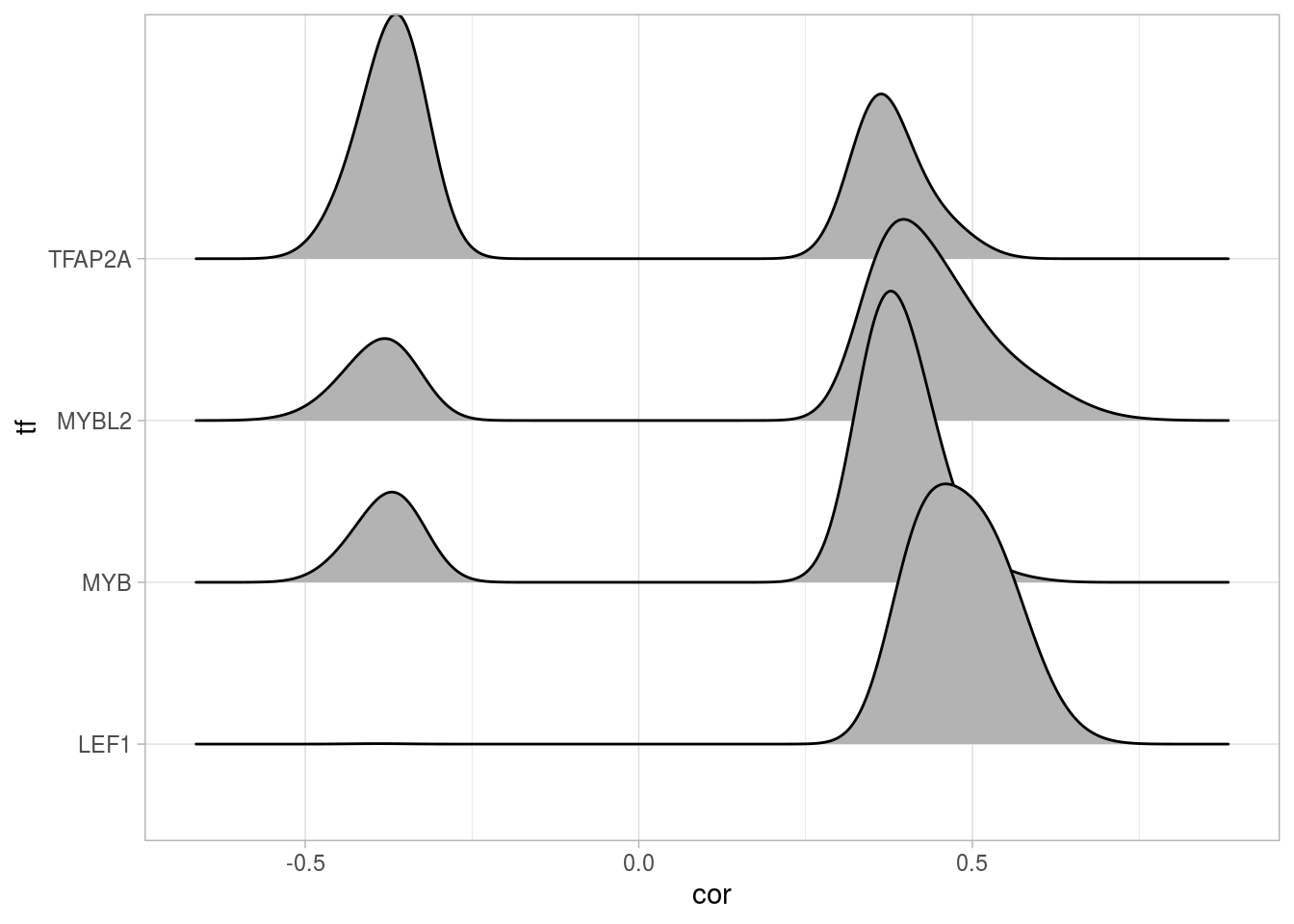``cor_upset(ob_tf)``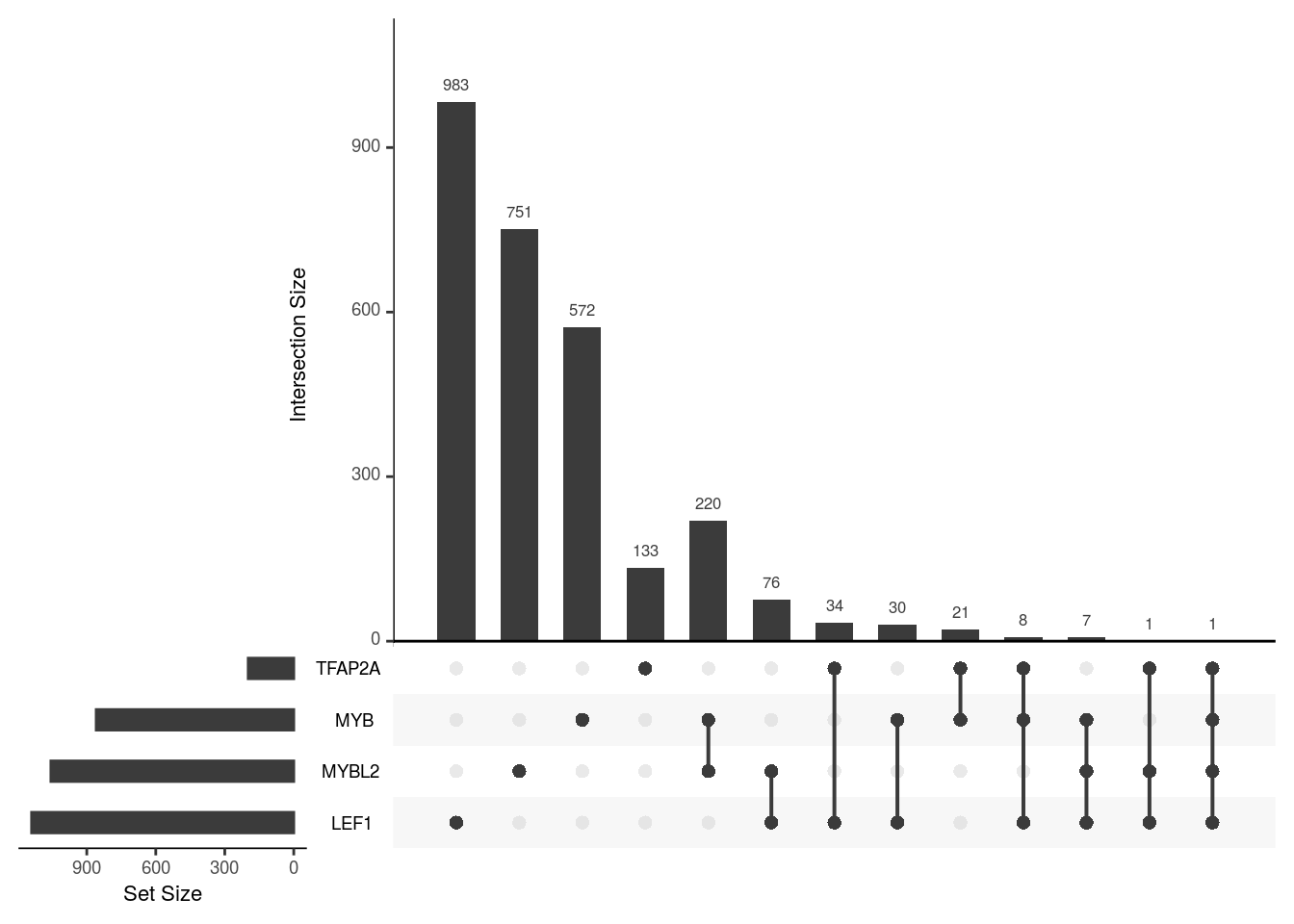## microRNA

Similarly, we use the output data.frame of microRNA-gene correlations in the STES study and summarize the numbers, densities and intersections using cRegulome.

``````# numbers of targets
table(creg_mir\$mirna_base)``````
``````##
##   hsa-let-7g   hsa-let-7i  hsa-mir-126 hsa-mir-130b hsa-mir-146a  hsa-mir-149
##          329          457           11          381          101          235
##  hsa-mir-150  hsa-mir-192 hsa-mir-193b  hsa-mir-195 hsa-mir-200a hsa-mir-200b
##           91          107          126          658          445          659
##  hsa-mir-202 hsa-mir-216b  hsa-mir-217  hsa-mir-221  hsa-mir-222  hsa-mir-27a
##          142            6            8          179          200          652
##  hsa-mir-27b  hsa-mir-30a  hsa-mir-30b  hsa-mir-30d   hsa-mir-31  hsa-mir-33a
##          608          746          540          507          209          205
##  hsa-mir-34a  hsa-mir-375  hsa-mir-378  hsa-mir-424  hsa-mir-431 hsa-mir-487b
##          196          110           54          395           37            3
##  hsa-mir-497  hsa-mir-92b  hsa-mir-99a  hsa-mir-99b
##          620          302           30           23``````
``````# construct a cmicroRNA object and plot
ob_mir <- cmicroRNA(creg_mir)
cor_joy(ob_mir)``````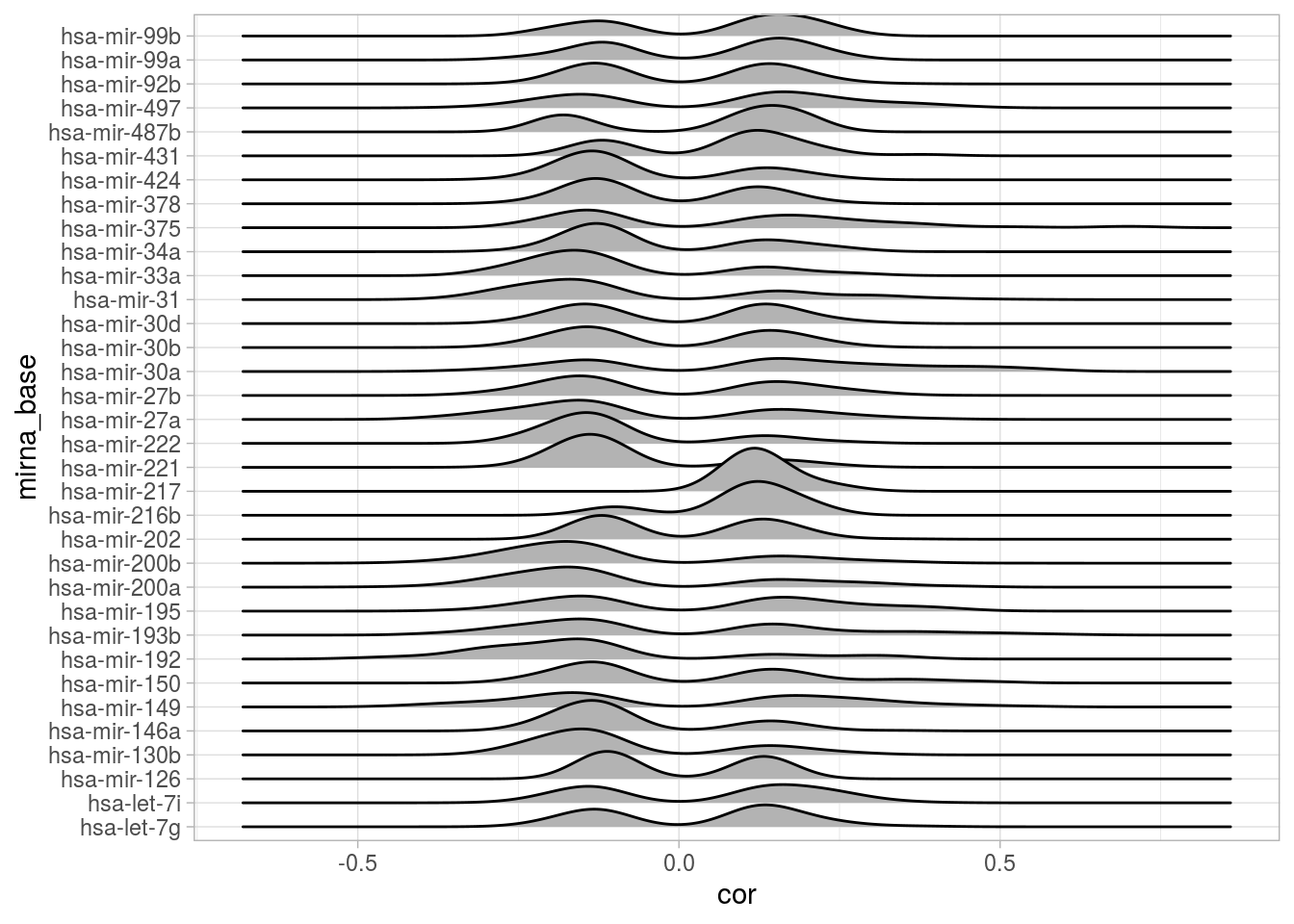``cor_upset(ob_mir)``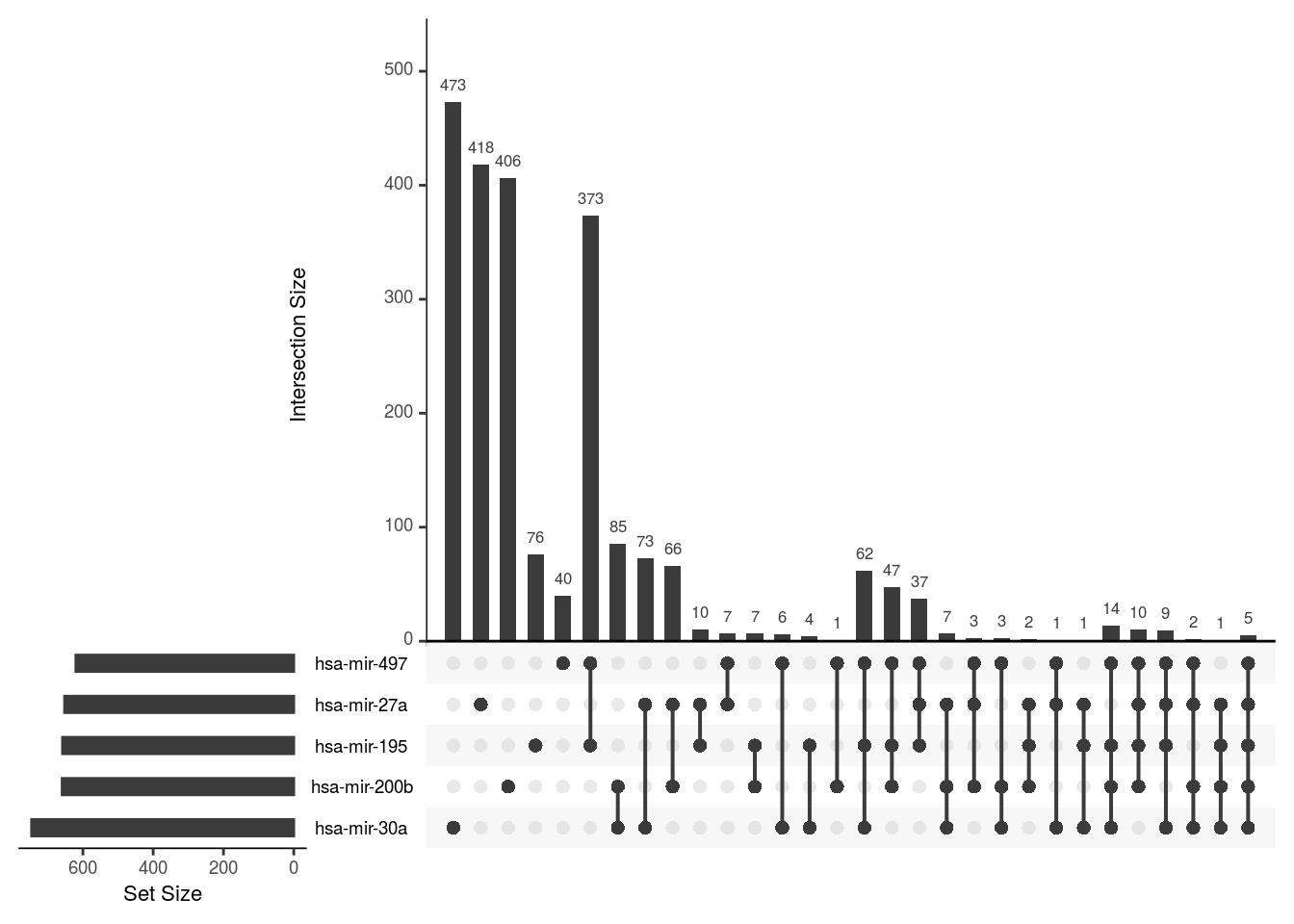## Network construction

For the purpose of constructing the network, we decided to limit the nodes to the TFs/microRNAs and gene targets with high correlation (absolute Pearson’s correlation > 0.3). We first return to `cRegulome` to query the database and tweak the output to be used with the `igraph` package to build the network.

``````# query cRegulome to get high correlated targets
creg_tf <- get_tf(conn,
tf = unique(tf\$SOURCE),
study = 'STES',
min_abs_cor = .3,
targets_only = TRUE)
creg_mir <- get_mir(conn,
mir = tolower(unique(mir\$AccID)),
study = 'STES',
min_abs_cor = .3,
targets_only = TRUE)``````

First, we construct two separate networks for the TF and the microRNA correlations using the `cor_igraph` function. Then, we combine the two networks and their attributes.

``````# make two separate networks
p1 <- cor_igraph(cTF(creg_tf))
p2 <- cor_igraph(cmicroRNA(creg_mir))

# combine networks
p <- graph.union(p1, p2)

# combine attributes
V(p)\$type[V(p)\$name %in% unique(creg_tf\$tf)] <- 'TF'
V(p)\$type[V(p)\$name %in% unique(creg_mir\$mirna_base)] <- 'microRNA'
V(p)\$type[is.na(V(p)\$type)] <- 'gene'

V(p)\$color <- c('lightgreen', 'blue', 'red')[factor(V(p)\$type)]

V(p)\$label <- ifelse(V(p)\$type == 'gene', '', V(p)\$name)

E(p)\$weight_1[is.na(E(p)\$weight_1)] <- E(p)\$weight_2[!is.na(E(p)\$weight_2)]``````

## Node degrees

Simple and useful information about the network can be obtained by analyzing the vertices `degree`. A node degree is the number of edges it shares with other nodes in the graph. Most of the nodes in the network we constructed have on edge/connection to another node. Most of the gene nodes has one edge and a few genes have 2 to 5 edges. Those are the ones that are regulated by two or more regulatory element (TF/microRNAs).

``````par(mfrow=c(1,2))
deg <- degree(p)

# full network degrees
plot(density(deg),
main = 'Full network degrees')

# gene degrees
plot(density(deg[V(p)\$type == 'gene']),
main = 'Gene nodes degrees')``````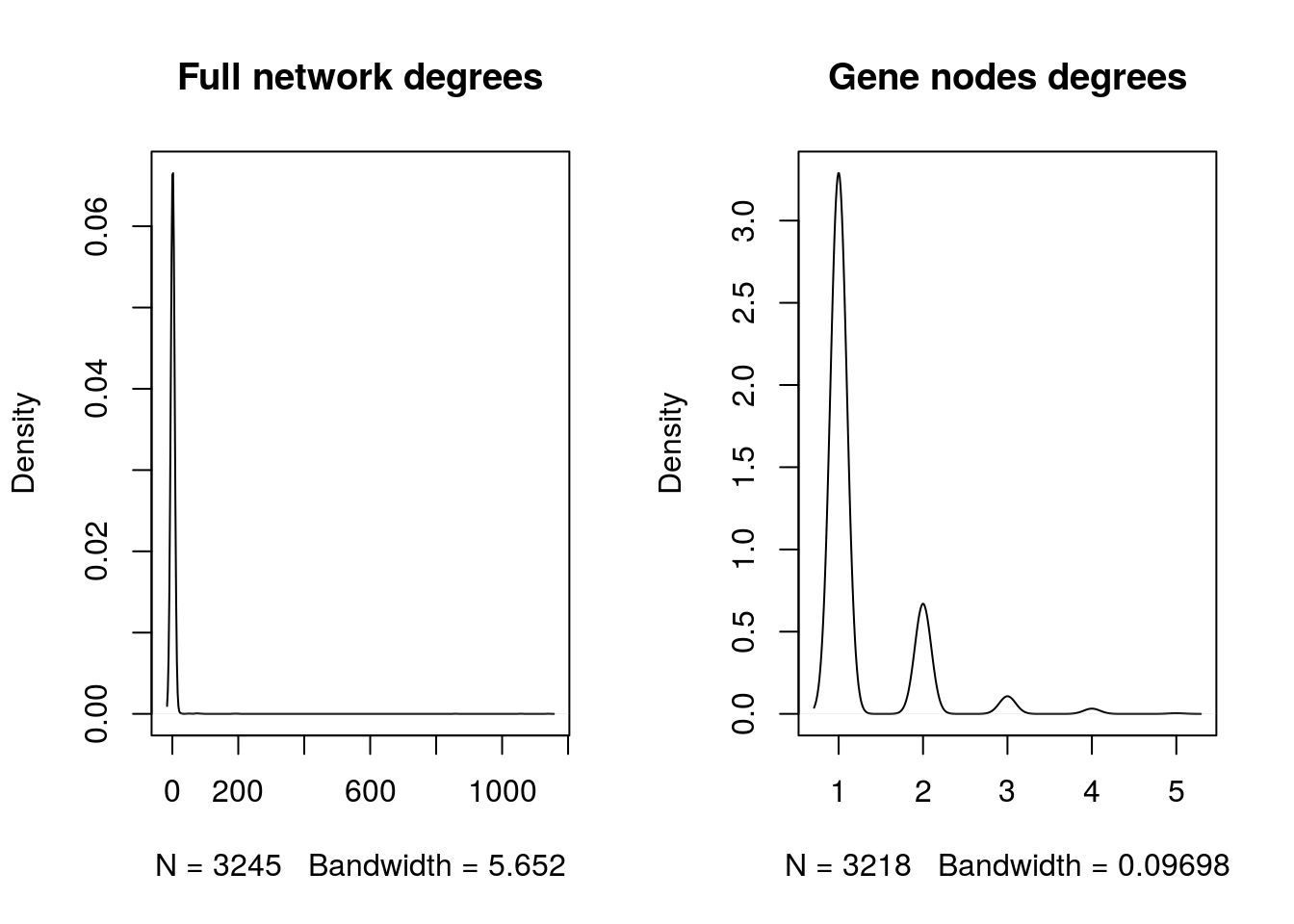Visualizing a dense network may not provide a lot of details, however we notice that the transcription factors (red) and the microRNAs (blue) are in many cases co-regulate one or more gene. So in the following section, we will used a clustering algorithm to capture these connections in sub-communities and used the KEGG enrichment analysis to ask whether they are biologically meaningful.

``````# plot network
set.seed(123)
par(mfrow=c(1,1))
new_p <- delete.vertices(p, deg < 2)
deg <- degree(new_p)
plot(new_p,
vertex.size = log2(deg)+1,
vertex.label.dist = .3,
vertex.label.cex   = .8,
vertex.label = V(new_p)\$label,
edge.arrow.size = 0)

legend('bottomleft',
legend = unique(V(new_p)\$type),
col = unique(V(new_p)\$color),
pch = 19)``````